# Chapter 8 Intro to Probability and Statistics Flashcards

Set Details Share
created 7 years ago by mmcausey
27,300 views
Chapter 8 Intro to Probability and Statistics
updated 7 years ago by mmcausey
Subjects:
mathematics
Page to share:
Embed this setcancel
COPY
code changes based on your size selection
Size:
X

1

Assume that a researcher wants to use sample data to test the claim that the sample is from a population with a standard deviation less than 1.8 min. The researcher will use a 0.05 significance level to test that claim. If the researcher wants to use the confidence interval method of testing​ hypotheses, what level of confidence should be used for the confidence​ interval? Will the conclusion based on the confidence interval be the same as the conclusion based on a hypothesis test that uses the​ P-value method or the critical value​ method?

a) If the researcher wants to use the confidence interval method of testing​ hypotheses, what level of confidence should be used for the confidence​ interval?

b) Will the conclusion based on the confidence interval be the same as the conclusion based on a hypothesis test that uses the​ P-value method or the critical value​ method?

a) Since this is a​ one-tailed test, the researcher should use a confidence level of 0.90.

b)Yes; the confidence interval method and the​ P-value or critical methods always lead to the same conclusion when the tested parameter is the standard deviation.

2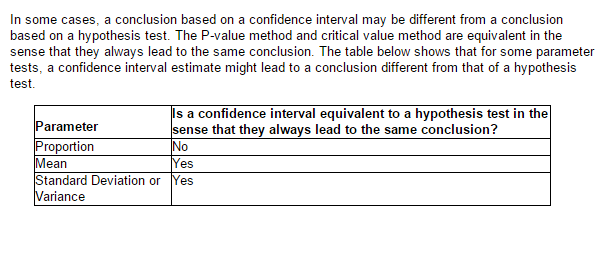Below is when the parameters have the same results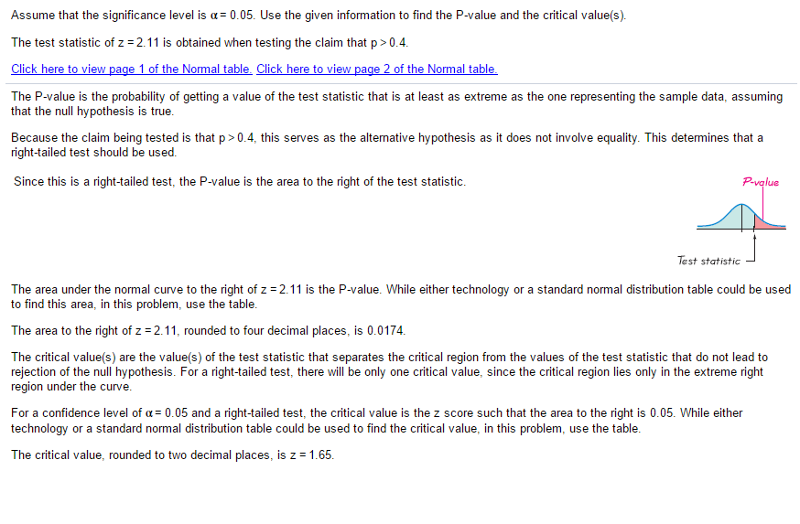See Below for Critical Z and P-Value from Z Score and significance for a right tailed test

3

Which of the following is NOT a requirement for testing a claim about a standard deviation or​ variance?

a) The population has a normal distribution.

b)The population must be skewed to the right.

c) The sample is a simple random sample.

d) The​ chi-square distribution is used.

The population must be skewed to the right.

4

Which of the following is NOT a property of the​ chi-square distribution?

a) The​ chi-square distribution is different for each number of degrees of​ freedom, df=n-1.

b) The​ chi-square distribution is not symmetric.

c) The mean of the​ chi-square distribution is 0.

d) The values of​ chi-square can be zero or​ positive, but they cannot be negative.

The mean of the​ chi-square distribution is 0.

5

Which of the following is not a requirement for testing a claim about a population with σ not​ known?

a) The value of the population standard deviation is not known.

b) The population​ mean, μ​, is equal to 1.

c) The sample is a simple random sample.

d) Either the population is normally distributed or n>30 or both.

The population​ mean, μ​, is equal to 1.

6

Which of the following is not a characteristic of the t​ test?

a) The Student t distribution has the same general bell shape as the standard normal distribution.

b) The t test is robust against a departure from normality.

c) The Student t distribution is different for different sample sizes.

d) The Student t distribution has a mean of t=0 and a standard deviation of s=1.

The Student t distribution has a mean of t=0 and a standard deviation of s=1.

7

Which of the following is not true when using the confidence interval method for testing a claim about μ when σ is​ unknown?

a) For a​ one-tailed hypothesis test with a 0.05 significance​ level, one must construct a​ 90% confidence interval.

b) The​ P-value method, the traditional​ method, and the confidence interval method are equivalent and yield the same results.

c) For a​ two-tailed hypothesis test with a 0.05 significance​ level, one must construct a​ 95% confidence interval.

d) The​ P-value method and the classical method are not equivalent to the confidence interval method in that they may yield different results.

The​ P-value method and the classical method are not equivalent to the confidence interval method in that they may yield different results.

8

Which of the following is NOT a requirement for testing a claim about a mean with σ ​known?

a) A conclusion based on a confidence interval estimate will be the same as a conclusion based on a hypothesis test.

b) If the sample results​ (or more extreme​ results) cannot easily occur when the null hypothesis is​ true, we explain the discrepancy between the assumption and the sample results by concluding that the assumption is​ true, so we do not reject the assumption.

c) If, under a given​ assumption, there is an exceptionally small probability of getting sample results at least as extreme as the results that were​ obtained, we conclude that the assumption is probably not correct.

d)
If the sample results​ (or more extreme​ results) can easily occur when the null hypothesis is​ true, we attribute the relatively small discrepancy between the assumption and the sample results to chance.

If the sample results​ (or more extreme​ results) cannot easily occur when the null hypothesis is​ true, we explain the discrepancy between the assumption and the sample results by concluding that the assumption is​ true, so we do not reject the assumption.

9

Which of the following is NOT a requirement of testing a claim about a population proportion using a formal method of hypothesis​ testing?

a) The conditions np>5 and nq>5 are both satisfied.

b) The sample observations are a simple random sample.

c) The lowercase​ symbol, p, represents the probability of getting a test statistic at least as extreme as the one representing sample data and is needed to test the claim.

d) The conditions for a binomial distribution are satisfied.

The lowercase​ symbol, p, represents the probability of getting a test statistic at least as extreme as the one representing sample data and is needed to test the claim.

10

Which of the following is NOT true when testing a claim about a​ proportion?

a) If you want to test a claim about population​ proportions, use the​ P-value method or the classical method of hypothesis testing.

b) A conclusion based on a confidence interval estimate will be the same as a conclusion based on a hypothesis test.

c) Both the traditional method and​ P-value method use the same standard deviation based on the claimed proportion​ p, but the confidence interval uses an estimated standard deviation based on the sample proportion p-hat.

d) When testing claims about population​ proportions, the traditional method and the​ P-value method are equivalent in the sense that they always yield the same result.

A conclusion based on a confidence interval estimate will be the same as a conclusion based on a hypothesis test.

11

​ _____________ is a procedure for testing a claim about a property of a population.

Hypothesis Test

12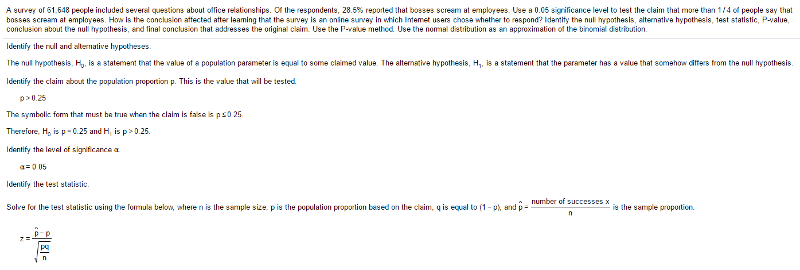Below Solves Z and P Value when X is expressed as a percentage of Sample size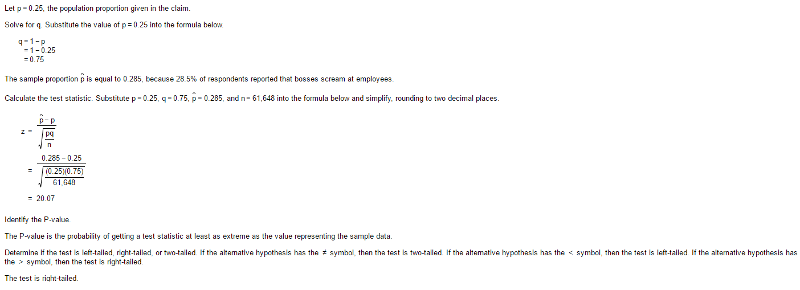Continued

13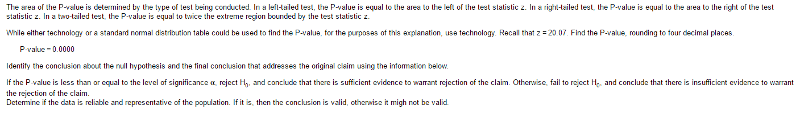Below Solves Z and P Value when X is expressed as a percentage of Sample size Part 3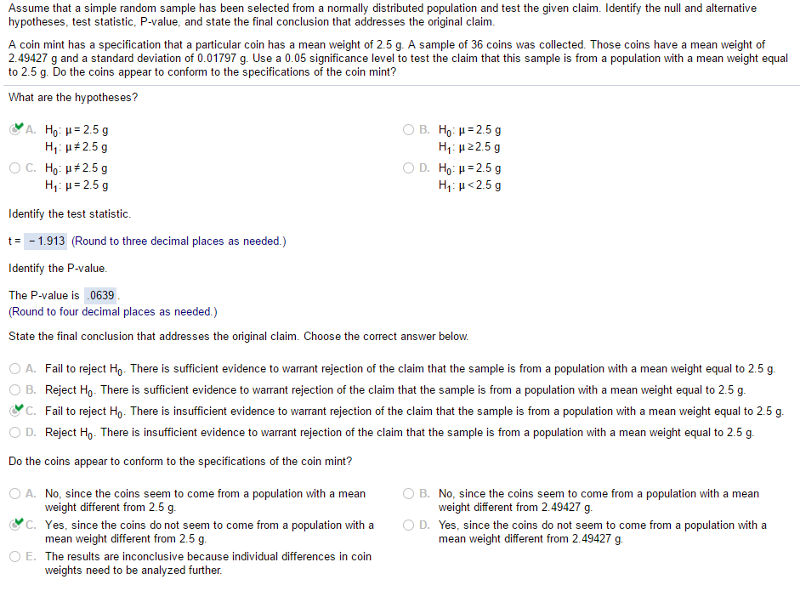Means Testing Finding T

14

The​ _____________ states that​ if, under a given​ assumption, the probability of a particular observed event is extremely​ small, we conclude that the assumption is probably not correct.

Rare Event Rule

15

Which of the following is NOT true of using the binomial probability distribution to test claims about a​ proportion?

a) This method uses the binomial probability distribution with the​ P-value method and uses the value of p assumed in the null hypothesis.

b) In a​ right-tailed test, the​ P-value is the probability of getting x or more successes among the n trials.

c) In a​ left-tailed test, the​ P-value is the probability of getting x or fewer successes among the n trials.

d) One requirement of this method is that np>5 and nq>5.

One requirement of this method is that np>5 and nq>5.

16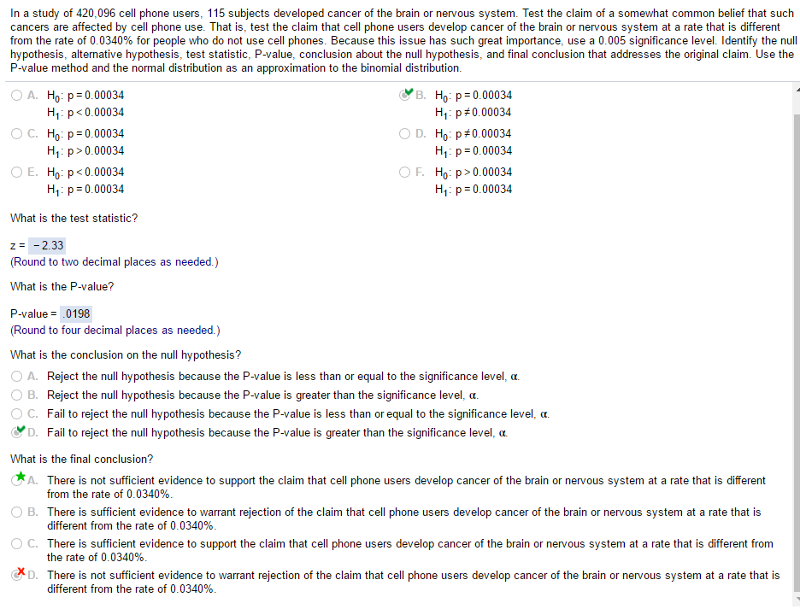P Not Equals

...